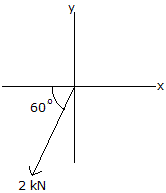# Engineering Mechanics - Force Vectors - Discussion

### Discussion :: Force Vectors - General Questions (Q.No.21)

21.Determine the magnitude of the x and y components of the 2—kN force.

 [A]. Fx = -1.414 kN, Fy = -1.414 kN [B]. Fx = -1.000 kN, Fy = -1.732 kN [C]. Fx = -1.732 kN, Fy = -1.000 kN [D]. Fx = -4.000 kN, Fy = -2.312 kN

Explanation:

No answer description available for this question.

 Renuka Batta said: (Jun 19, 2012) In the x-direction the angle is 120 therefore fx=2*cos (180-60) =-1.000kN, fy=2*sin (180+60) =-1.732kN.

 Yoseph said: (Dec 24, 2013) But if we use instead of it as. Fx = -2kNcos60 and Fy = -2kNsin60 then it will be, Fx = -1.732kN and Fy = -1.000kN.